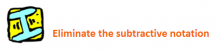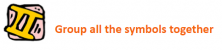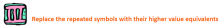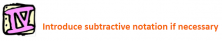Smartick is a fun way to learn math!Mar25

# Roman Numerals: Do You Know How to Add Them?

In this entry, we are going to learn to complete operations with Roman numerals. It is important to refresh our knowledge about these numbers before we begin.

The Roman numeral system is very different from the decimal system that we use on a daily basis. Look closely at the numbers 21 and 12, they are different in value but we use the same symbols to write them. This is because the decimal system is positional, meaning the value of the symbol depends on the position it occupies. However, this is not the case with Roman numerals. The symbol ‘I’ always has a value of 1 independent from whatever position it appears in. Therefore, we say that the Roman numeral system is additive, meaning each symbol represents the same value that is added to the previous one.

With this in mind, we can now ask ourselves how to add two Roman numerals. The solution that is usually taught is ”translating” Roman numerals into the decimal system, completing the operation, and then changing the numbers back to Roman numerals. But this doesn’t teach us anything new, so we are going to present a method that uses the additivity of the Roman numeral system in order to add without changing numbers to the decimal system.

### Method for adding Roman numerals

Let’s imagine that we are adding the numbers CXXIV and CXL, but we have no idea how to interpret them in the decimal system. No problem, our method helps us find the right solution. Let’s go!As you know, when a symbol of a lesser value appears to the left of another, they are subtracted. This is known as subtractive notation. In this first stage of the method, we need to eliminate including any necessary symbols. For example, IV becomes IIII. In our case, CXXIV  → CXXIIII and CXL → CXXXX.In this step, we gather all the symbols that we have obtained from the previous step and order them from greatest to least, therefore CXXIIII y CXXXX → CCXXXXXXIIIIIn our addition problem we have six X’s, so we can change 5 X’s for an L. This way we have CCXXXXXXIIII → CCLXIIIIWe review the sequence that we have done, making sure it follows the rules for writing Roman numerals and if not, then we make the necessary modifications. So we have to modify the last four I’s and change them to IV… which gives us CCLXIIII → CCLXIV

Now we have found the sum of  CXXIV and CXL:  CCLXIV! Notice that, as we promised, we did not have to change any numbers to the decimal system. Check to see that the answer is correct!

I hope that you have enjoyed this post and that you learned something new about Roman numerals. If you want to continue learning more about elementary math, login to Smartick what are you waiting for?